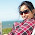# for Loop - Basic Java Tutorials For Selenium WebDriver

We have learnt different if else condition statements in my previous post. Now let we move to loops in java software development language. Loops(for loop, while loop, do while loop) have very important role in selenium webdriver software test case development with java or any other languages. For loop in selenium is widely used. As you know, sometimes you need to perform same action multiple times(Example : 100 or more times) on your web page. Now if you will write multiple lines of code to perform same action multiple times then it will increase your code size. For the best practice, you need to use for loop in selenium java in this kind of situations.

Let me describe you selenium java for loop in this post and you can view my NEXT POST for reading about while loop.

### for loop in selenium java

There are three parts inside for loop of java software development language. 1. Variable Initialization, 2. Condition To Terminate and 3. Increment/Decrements variable. for loop will be terminated when condition to terminate will becomes false.

### Example of for loop in selenium :

``````for(int i=0; i<=3; i++){
System.out.println("Value Of Variable i is " +i);
}``````

Bellow given example is simple example of for loop in selenium java. run it in your eclipse and verify the result.
``````public class forloop {

public static void main(String[] args) {
for(int i=0; i<=3; i++){ //This loop will be executed 4 times
System.out.println("Value Of Variable i is " +i);
}

System.out.println("");
int i=0;
int k = 200;
for(int j=3; j>=i; j--){ //This loop will be executed 4 times
System.out.println("Value Of Variable j is " +j);
k = k-10;
}
System.out.println("");
System.out.println("Value Of Variable k is " +k);

}

}``````

When you will run above given example, you will get bellow given output.
``````Value Of Variable i is 0
Value Of Variable i is 1
Value Of Variable i is 2
Value Of Variable i is 3

Value Of Variable j is 3
Value Of Variable j is 2
Value Of Variable j is 1
Value Of Variable j is 0

Value Of Variable k is 160``````

1.Very Useful. Thank You

2.What is the difference between for and while loop.

1.for loop:is used for running the loop for more than once and while loop is only for one time,checking the condition.

3.why k is 160 ? please explain

1.Place System.out.println("Value Of Variable k is " +k); under k = k-10; and see the result :)

2.no the answer is 170 , because at third step , j decrements to 1 and i increments to 2 ,1>=2 which is false , hence loop terminates at this step

4.@sunrise487 K is initialised as 200 and the second loop is executed 4 times so k is decreased by 10 4 times so 160.

5.the initial value of k is 200 and in the first iteration it checks whether int j=3; j>=i ; YES. so it executes the below statements inside the for loop
system.out.print (j); then k=k-10 ; ie k=200-10; now at the end of first iteration k holds the value 190 (200-10). similarly after 4 iteration k value get decreased to 160 (10 will be minus in each iteration and once the condition fails it will come out of the loop and print the value of k which is 160)

6.very good discription. thanks

7.Very good discription . thanks

8.This blog is excellent for selenium, can you please share some more examples on all topics

9.Very well explained !!!
To see how iteration works, just add the below statement as shown below:
After k = k - 10; add the below print line:

System.out.println("value of k is "+k);

Result:Value of j is 3
value of k is 190
Value of j is 2
value of k is 180
Value of j is 1
value of k is 170
Value of j is 0
value of k is 160

10.what if the second For loop is written this way.
System.out.println("");
int i=0;
int k = 200;
for(int j=3; j>=i; --j){ //This loop will be executed 4 times
System.out.println("Value Of Variable j is " +j);
k = k-10;
}
System.out.println("");
System.out.println("Value Of Variable k is " +k);

11.Add this code and run it. it will show the step by step results:
System.out.println("");
int i=0;
int k = 200;
for(int j=3; j>=i; j--){ //This loop will be executed 4 times
System.out.println("Value Of Variable j is " +j);
k = k-10;
System.out.println("Value Of Variable k is " +k);
}